GFG App
Open AppBrowser
Continue

# Menu driven program for all operations on singly linked list in C

A Linked List is a linear data structure that consists of two parts: one is the data part and the other is the address part. In this article, all the common operations of a singly linked list are discussed in one menu-driven program.

Operations to be performed:

• createList(): To create the list with the ‘n’ number of nodes initially as defined by the user.
• traverse(): To see the contents of the linked list, it is necessary to traverse the given linked list. The given traverse() function traverses and prints the content of the linked list.
• insertAtFront(): This function simply inserts an element at the front/beginning of the linked list.
• insertAtEnd(): This function inserts an element at the end of the linked list.
• insertAtPosition(): This function inserts an element at a specified position in the linked list.
• deleteFirst(): This function simply deletes an element from the front/beginning of the linked list.
• deleteEnd(): This function simply deletes an element from the end of the linked list.
• deletePosition(): This function deletes an element from a specified position in the linked list.
• maximum(): This function finds the maximum element in a linked list.
• mean(): This function finds the mean of the elements in a linked list.
• sort(): This function sorts the given linked list in ascending order.
• reverseLL(): This function reverses the given linked list.
• display(): This function displays the linked list.
• search(): This function searches for an element user wants to search.

Below is the implementation of the above operations:

## C

 `// C program for the all operations in` `// the Singly Linked List`   `#include ` `#include `   `// Linked List Node` `struct` `node {` `    ``int` `info;` `    ``struct` `node* link;` `};` `struct` `node* start = NULL;`   `// Function to create list with n nodes initially` `void` `createList()` `{` `    ``if` `(start == NULL) {` `        ``int` `n;` `        ``printf``(``"\nEnter the number of nodes: "``);` `        ``scanf``(``"%d"``, &n);` `        ``if` `(n != 0) {` `            ``int` `data;` `            ``struct` `node* newnode;` `            ``struct` `node* temp;` `            ``newnode = ``malloc``(``sizeof``(``struct` `node));` `            ``start = newnode;` `            ``temp = start;` `            ``printf``(``"\nEnter number to"` `                   ``" be inserted : "``);` `            ``scanf``(``"%d"``, &data);` `            ``start->info = data;`   `            ``for` `(``int` `i = 2; i <= n; i++) {` `                ``newnode = ``malloc``(``sizeof``(``struct` `node));` `                ``temp->link = newnode;` `                ``printf``(``"\nEnter number to"` `                       ``" be inserted : "``);` `                ``scanf``(``"%d"``, &data);` `                ``newnode->info = data;` `                ``temp = temp->link;` `            ``}` `        ``}` `        ``printf``(``"\nThe list is created\n"``);` `    ``}` `    ``else` `        ``printf``(``"\nThe list is already created\n"``);` `}`   `// Function to traverse the linked list` `void` `traverse()` `{` `    ``struct` `node* temp;`   `    ``// List is empty` `    ``if` `(start == NULL)` `        ``printf``(``"\nList is empty\n"``);`   `    ``// Else print the LL` `    ``else` `{` `        ``temp = start;` `        ``while` `(temp != NULL) {` `            ``printf``(``"Data = %d\n"``, temp->info);` `            ``temp = temp->link;` `        ``}` `    ``}` `}`   `// Function to insert at the front` `// of the linked list` `void` `insertAtFront()` `{` `    ``int` `data;` `    ``struct` `node* temp;` `    ``temp = ``malloc``(``sizeof``(``struct` `node));` `    ``printf``(``"\nEnter number to"` `           ``" be inserted : "``);` `    ``scanf``(``"%d"``, &data);` `    ``temp->info = data;`   `    ``// Pointer of temp will be` `    ``// assigned to start` `    ``temp->link = start;` `    ``start = temp;` `}`   `// Function to insert at the end of` `// the linked list` `void` `insertAtEnd()` `{` `    ``int` `data;` `    ``struct` `node *temp, *head;` `    ``temp = ``malloc``(``sizeof``(``struct` `node));`   `    ``// Enter the number` `    ``printf``(``"\nEnter number to"` `           ``" be inserted : "``);` `    ``scanf``(``"%d"``, &data);`   `    ``// Changes links` `    ``temp->link = 0;` `    ``temp->info = data;` `    ``head = start;` `    ``while` `(head->link != NULL) {` `        ``head = head->link;` `    ``}` `    ``head->link = temp;` `}`   `// Function to insert at any specified` `// position in the linked list` `void` `insertAtPosition()` `{` `    ``struct` `node *temp, *newnode;` `    ``int` `pos, data, i = 1;` `    ``newnode = ``malloc``(``sizeof``(``struct` `node));`   `    ``// Enter the position and data` `    ``printf``(``"\nEnter position and data :"``);` `    ``scanf``(``"%d %d"``, &pos, &data);`   `    ``// Change Links` `    ``temp = start;` `    ``newnode->info = data;` `    ``newnode->link = 0;` `    ``while` `(i < pos - 1) {` `        ``temp = temp->link;` `        ``i++;` `    ``}` `    ``newnode->link = temp->link;` `    ``temp->link = newnode;` `}`   `// Function to delete from the front` `// of the linked list` `void` `deleteFirst()` `{` `    ``struct` `node* temp;` `    ``if` `(start == NULL)` `        ``printf``(``"\nList is empty\n"``);` `    ``else` `{` `        ``temp = start;` `        ``start = start->link;` `        ``free``(temp);` `    ``}` `}`   `// Function to delete from the end` `// of the linked list` `void` `deleteEnd()` `{` `    ``struct` `node *temp, *prevnode;` `    ``if` `(start == NULL)` `        ``printf``(``"\nList is Empty\n"``);` `    ``else` `{` `        ``temp = start;` `        ``while` `(temp->link != 0) {` `            ``prevnode = temp;` `            ``temp = temp->link;` `        ``}` `        ``free``(temp);` `        ``prevnode->link = 0;` `    ``}` `}`   `// Function to delete from any specified` `// position from the linked list` `void` `deletePosition()` `{` `    ``struct` `node *temp, *position;` `    ``int` `i = 1, pos;`   `    ``// If LL is empty` `    ``if` `(start == NULL)` `        ``printf``(``"\nList is empty\n"``);`   `    ``// Otherwise` `    ``else` `{` `        ``printf``(``"\nEnter index : "``);`   `        ``// Position to be deleted` `        ``scanf``(``"%d"``, &pos);` `        ``position = ``malloc``(``sizeof``(``struct` `node));` `        ``temp = start;`   `        ``// Traverse till position` `        ``while` `(i < pos - 1) {` `            ``temp = temp->link;` `            ``i++;` `        ``}`   `        ``// Change Links` `        ``position = temp->link;` `        ``temp->link = position->link;`   `        ``// Free memory` `        ``free``(position);` `    ``}` `}`   `// Function to find the maximum element` `// in the linked list` `void` `maximum()` `{` `    ``int` `a;` `    ``int` `i;` `    ``struct` `node* temp;`   `    ``// If LL is empty` `    ``if` `(start == NULL)` `        ``printf``(``"\nList is empty\n"``);`   `    ``// Otherwise` `    ``else` `{` `        ``temp = start;` `        ``int` `max = temp->info;`   `        ``// Traverse LL and update the` `        ``// maximum element` `        ``while` `(temp != NULL) {`   `            ``// Update the maximum` `            ``// element` `            ``if` `(max < temp->info)` `                ``max = temp->info;` `            ``temp = temp->link;` `        ``}` `        ``printf``(``"\nMaximum number "` `               ``"is : %d "``,` `               ``max);` `    ``}` `}`   `// Function to find the mean of the` `// elements in the linked list` `void` `mean()` `{` `    ``int` `a;` `    ``int` `i;` `    ``struct` `node* temp;`   `    ``// If LL is empty` `    ``if` `(start == NULL)` `        ``printf``(``"\nList is empty\n"``);`   `    ``// Otherwise` `    ``else` `{` `        ``temp = start;`   `        ``// Stores the sum and count of` `        ``// element in the LL` `        ``int` `sum = 0, count = 0;` `        ``float` `m;`   `        ``// Traverse the LL` `        ``while` `(temp != NULL) {`   `            ``// Update the sum` `            ``sum = sum + temp->info;` `            ``temp = temp->link;` `            ``count++;` `        ``}`   `        ``// Find the mean` `        ``m = sum / count;`   `        ``// Print the mean value` `        ``printf``(``"\nMean is %f "``, m);` `    ``}` `}`   `// Function to sort the linked list` `// in ascending order` `void` `sort()` `{` `    ``struct` `node* current = start;` `    ``struct` `node* index = NULL;` `    ``int` `temp;`   `    ``// If LL is empty` `    ``if` `(start == NULL) {` `        ``return``;` `    ``}`   `    ``// Else` `    ``else` `{`   `        ``// Traverse the LL` `        ``while` `(current != NULL) {` `            ``index = current->link;`   `            ``// Traverse the LL nestedly` `            ``// and find the minimum` `            ``// element` `            ``while` `(index != NULL) {`   `                ``// Swap with it the value` `                ``// at current` `                ``if` `(current->info > index->info) {` `                    ``temp = current->info;` `                    ``current->info = index->info;` `                    ``index->info = temp;` `                ``}` `                ``index = index->link;` `            ``}`   `            ``// Update the current` `            ``current = current->link;` `        ``}` `    ``}` `}`   `// Function to reverse the linked list` `void` `reverseLL()` `{` `    ``struct` `node *t1, *t2, *temp;` `    ``t1 = t2 = NULL;`   `    ``// If LL is empty` `    ``if` `(start == NULL)` `        ``printf``(``"List is empty\n"``);`   `    ``// Else` `    ``else` `{`   `        ``// Traverse the LL` `        ``while` `(start != NULL) {`   `            ``// reversing of points` `            ``t2 = start->link;` `            ``start->link = t1;` `            ``t1 = start;` `            ``start = t2;` `        ``}` `        ``start = t1;`   `        ``// New head Node` `        ``temp = start;`   `        ``printf``(``"Reversed linked "` `               ``"list is : "``);`   `        ``// Print the LL` `        ``while` `(temp != NULL) {` `            ``printf``(``"%d "``, temp->info);` `            ``temp = temp->link;` `        ``}` `    ``}` `}`   `// Function to search an element in linked list` `void` `search()` `{` `    ``int` `found = -1;` `    ``// creating node to traverse` `    ``struct` `node* tr = start;`   `    ``// first checking if the list is empty or not` `    ``if` `(start == NULL) {` `        ``printf``(``"Linked list is empty\n"``);` `    ``}` `    ``else` `{` `        ``printf``(``"\nEnter the element you want to search: "``);` `        ``int` `key;` `        ``scanf``(``"%d"``, &key);`   `        ``// checking by traversing` `        ``while` `(tr != NULL) {` `            ``// checking for key` `            ``if` `(tr->info == key) {` `                ``found = 1;` `                ``break``;` `            ``}` `            ``// moving forward if not at this position` `            ``else` `{` `                ``tr = tr->link;` `            ``}` `        ``}`   `        ``// printing found or not` `        ``if` `(found == 1) {` `            ``printf``(` `                ``"Yes, %d is present in the linked list.\n"``,` `                ``key);` `        ``}` `        ``else` `{` `            ``printf``(``"No, %d is not present in the linked "` `                   ``"list.\n"``,` `                   ``key);` `        ``}` `    ``}` `}`   `// Driver Code` `int` `main()` `{` `    ``int` `choice;` `    ``while` `(1) {`   `        ``printf``(``"\n\t1  To see list\n"``);` `        ``printf``(``"\t2  For insertion at"` `               ``" starting\n"``);` `        ``printf``(``"\t3  For insertion at"` `               ``" end\n"``);` `        ``printf``(``"\t4  For insertion at "` `               ``"any position\n"``);` `        ``printf``(``"\t5  For deletion of "` `               ``"first element\n"``);` `        ``printf``(``"\t6  For deletion of "` `               ``"last element\n"``);` `        ``printf``(``"\t7  For deletion of "` `               ``"element at any position\n"``);` `        ``printf``(``"\t8  To find maximum among"` `               ``" the elements\n"``);` `        ``printf``(``"\t9  To find mean of "` `               ``"the elements\n"``);` `        ``printf``(``"\t10 To sort element\n"``);` `        ``printf``(``"\t11 To reverse the "` `               ``"linked list\n"``);` `        ``printf``(``"\t12 Search an element in linked list\n"``);` `        ``printf``(``"\t13 To exit\n"``);` `        ``printf``(``"\nEnter Choice :\n"``);` `        ``scanf``(``"%d"``, &choice);`   `        ``switch` `(choice) {` `        ``case` `1:` `            ``traverse();` `            ``break``;` `        ``case` `2:` `            ``insertAtFront();` `            ``break``;` `        ``case` `3:` `            ``insertAtEnd();` `            ``break``;` `        ``case` `4:` `            ``insertAtPosition();` `            ``break``;` `        ``case` `5:` `            ``deleteFirst();` `            ``break``;` `        ``case` `6:` `            ``deleteEnd();` `            ``break``;` `        ``case` `7:` `            ``deletePosition();` `            ``break``;` `        ``case` `8:` `            ``maximum();` `            ``break``;` `        ``case` `9:` `            ``mean();` `            ``break``;` `        ``case` `10:` `            ``sort();` `            ``break``;` `        ``case` `11:` `            ``reverseLL();` `            ``break``;` `        ``case` `12:` `            ``search();` `            ``break``;` `        ``case` `13:` `            ``exit``(1);` `            ``break``;` `        ``default``:` `            ``printf``(``"Incorrect Choice\n"``);` `        ``}` `    ``}` `    ``return` `0;` `}`

Output: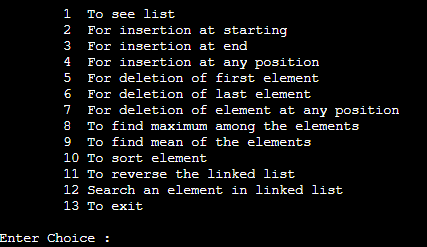Insertion at the starting: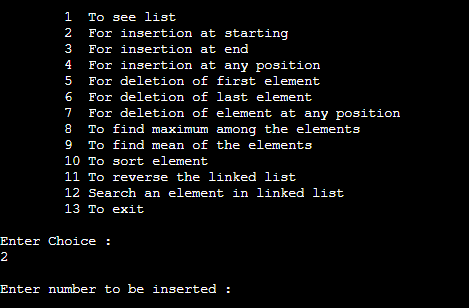Insertion at the end: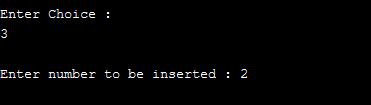Insertion at specific position: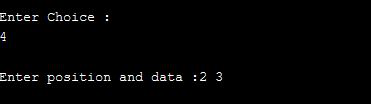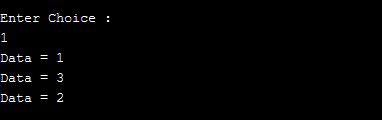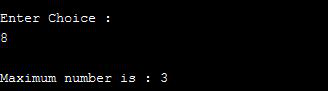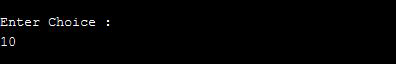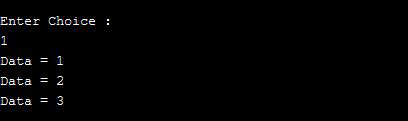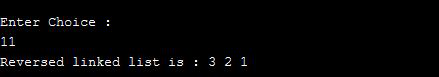Delete the first and last element with choice 5 and 6: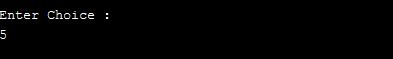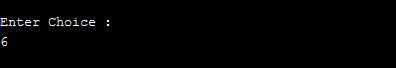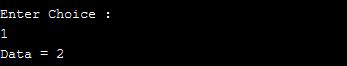Searching the element with choice 12: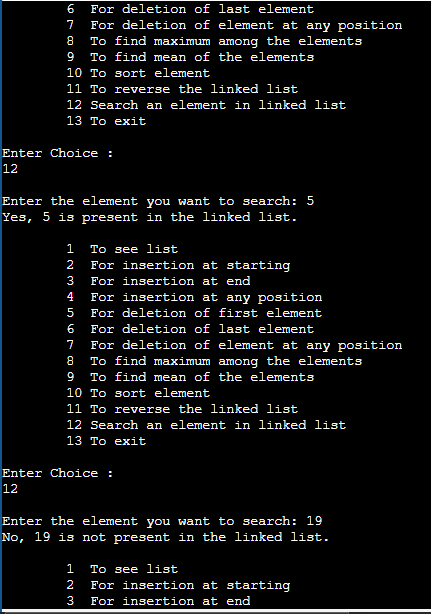My Personal Notes arrow_drop_up